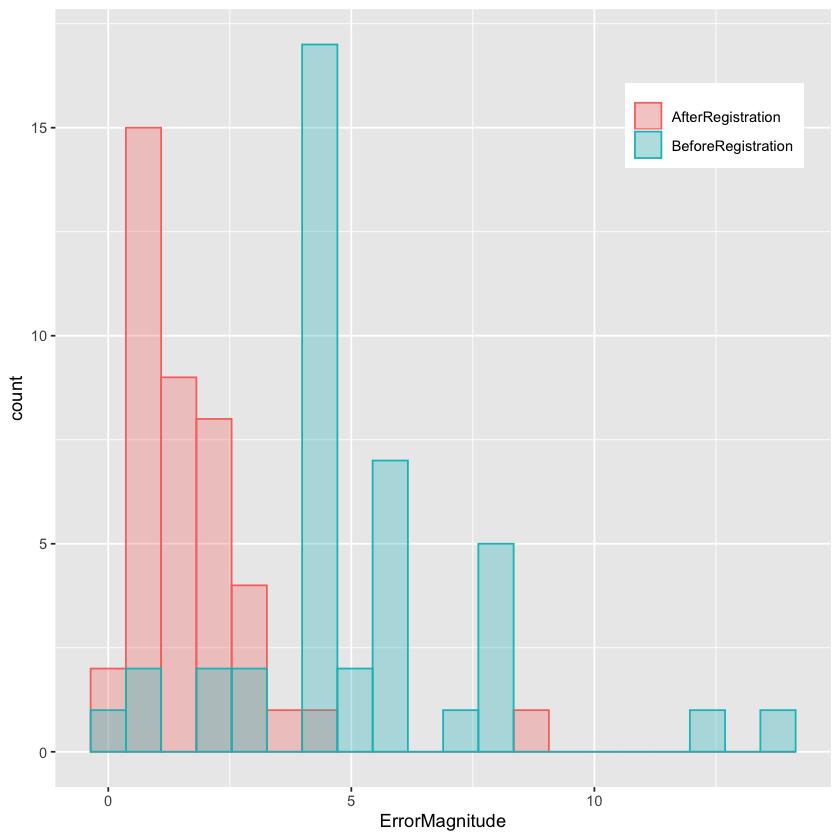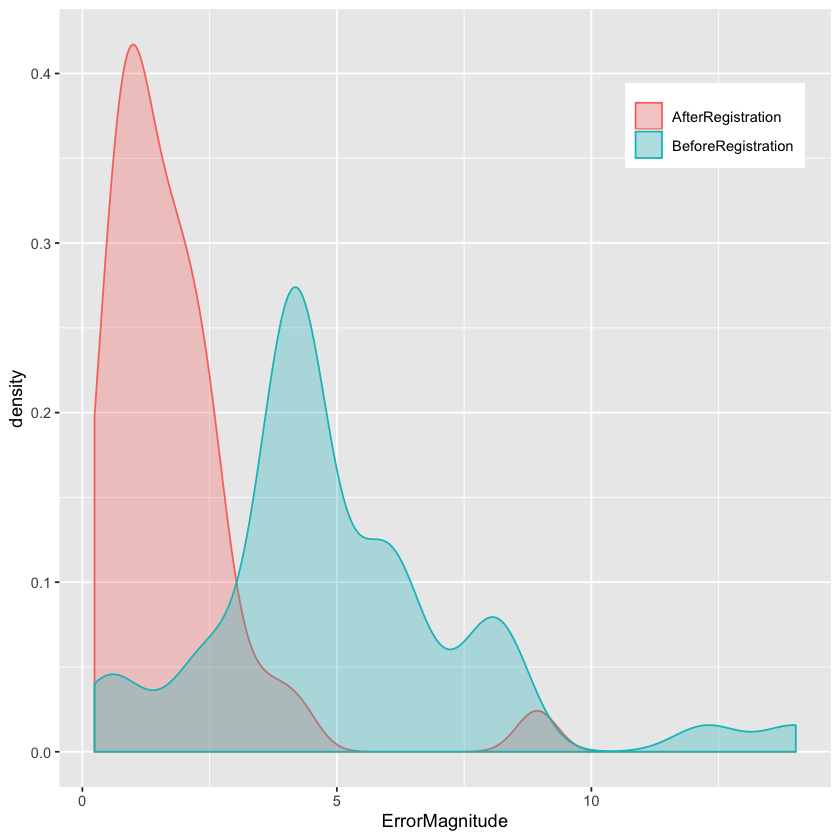# Non-Rigid Registration: Demons

This notebook illustrates the use of the Demons based non-rigid registration set of algorithms in SimpleITK. These include both the DemonsMetric which is part of the registration framework and Demons registration filters which are not.

The data we work with is a 4D (3D+time) thoracic-abdominal CT, the Point-validated Pixel-based Breathing Thorax Model (POPI) model. This data consists of a set of temporal CT volumes, a set of masks segmenting each of the CTs to air/body/lung, and a set of corresponding points across the CT volumes.

The POPI model is provided by the Léon Bérard Cancer Center & CREATIS Laboratory, Lyon, France. The relevant publication is:

J. Vandemeulebroucke, D. Sarrut, P. Clarysse, "The POPI-model, a point-validated pixel-based breathing thorax model", Proc. XVth International Conference on the Use of Computers in Radiation Therapy (ICCR), Toronto, Canada, 2007.

The POPI data, and additional 4D CT data sets with reference points are available from the CREATIS Laboratory here.

In :
library(SimpleITK)

# If the environment variable SIMPLE_ITK_MEMORY_CONSTRAINED_ENVIRONMENT is set, this will override the ReadImage
# function so that it also resamples the image to a smaller size (testing environment is memory constrained).
source("setup_for_testing.R")

library(ggplot2)
library(tidyr)
library(purrr)
# Utility method that either downloads data from the Girder repository or

Loading required package: rPython



## Utilities¶

Utility methods used in the notebook for display and registration evaluation.

In :
source("registration_utilities.R")


Load all of the images, masks and point data into corresponding lists. If the data is not available locally it will be downloaded from the original remote repository.

Take a look at a temporal slice for a specific coronal index (center of volume). According to the documentation on the POPI site, volume number one corresponds to end inspiration (maximal air volume).

You can modify the coronal index to look at other temporal slices.

In :
body_label <- 0
air_label <- 1
lung_label <- 2

image_file_names <- file.path("POPI", "meta", paste0(0:9, "0-P.mhd"))
# Read the CT images as 32bit float, the pixel type required for registration.
image_list <- lapply(image_file_names, function(image_file_name) ReadImage(fetch_data(image_file_name), "sitkFloat32"))

points_file_names <- file.path("POPI", "landmarks", paste0(0:9, "0-Landmarks.pts"))

# Look at a temporal slice for the specific coronal index
coronal_index <- as.integer(round(image_list[]$GetHeight()/2.0)) temporal_slice <- temporal_coronal_with_overlay(coronal_index, image_list, mask_list, lung_label, -1024, 976) # Flip the image so that it corresponds to the standard radiological display. Show(temporal_slice[,seq(temporal_slice$GetHeight(),0,-1),])


## Demons Registration¶

This function will align the fixed and moving images using the Demons registration method. If given a mask, the similarity metric will be evaluated using points sampled inside the mask. If given fixed and moving points the similarity metric value and the target registration errors will be displayed during registration.

As this notebook performs intra-modal registration, we can readily use the Demons family of algorithms.

We start by using the registration framework with SetMetricAsDemons. We use a multiscale approach which is readily available in the framework. We then illustrate how to use the Demons registration filters that are not part of the registration framework.

In :
demons_registration <- function(fixed_image, moving_image)
{
registration_method <- ImageRegistrationMethod()

# Create initial identity transformation.
transform_to_displacment_field_filter <- TransformToDisplacementFieldFilter()
transform_to_displacment_field_filter$SetReferenceImage(fixed_image) # The image returned from the initial_transform_filter is transferred to the transform and cleared out. initial_transform <- DisplacementFieldTransform(transform_to_displacment_field_filter$Execute(Transform()))

# Regularization (update field - viscous, total field - elastic).
initial_transform$SetSmoothingGaussianOnUpdate(varianceForUpdateField=0.0, varianceForTotalField=2.0) registration_method$SetInitialTransform(initial_transform)

registration_method$SetMetricAsDemons(10) #intensities are equal if the difference is less than 10HU # Multi-resolution framework. registration_method$SetShrinkFactorsPerLevel(shrinkFactors = c(4,2,1))
registration_method$SetSmoothingSigmasPerLevel(smoothingSigmas = c(8,4,0)) registration_method$SetInterpolator("sitkLinear")
# If you have time, run this code using the ConjugateGradientLineSearch, otherwise run as is.
#registration_method$SetOptimizerAsConjugateGradientLineSearch(learningRate=1.0, numberOfIterations=20, convergenceMinimumValue=1e-6, convergenceWindowSize=10) registration_method$SetOptimizerAsGradientDescent(learningRate=1.0, numberOfIterations=20, convergenceMinimumValue=1e-6, convergenceWindowSize=10)
registration_method$SetOptimizerScalesFromPhysicalShift() return (registration_method$Execute(fixed_image, moving_image))
}


Running the Demons registration with the conjugate gradient optimizer on this data takes a long time which is why the code above uses gradient descent. If you are more interested in accuracy and have the time then switch to the conjugate gradient optimizer.

In :
# Select the fixed and moving images, valid entries are in [1,10]
fixed_image_index <- 1
moving_image_index <- 8

tx <- demons_registration(fixed_image = image_list[[fixed_image_index]],
moving_image = image_list[[moving_image_index]])

initial_errors <- registration_errors(Euler3DTransform(), points_list[[fixed_image_index]], points_list[[moving_image_index]])
final_errors <- registration_errors(tx, points_list[[fixed_image_index]], points_list[[moving_image_index]])

# Plot the TRE histograms before and after registration.
df <- data.frame(AfterRegistration=final_errors, BeforeRegistration=initial_errors)
df.long <- gather(df, key=ErrorType, value=ErrorMagnitude)

ggplot(df.long, aes(x=ErrorMagnitude, group=ErrorType, colour=ErrorType, fill=ErrorType)) +
geom_histogram(bins=20,position='identity', alpha=0.3) +
theme(legend.title=element_blank(), legend.position=c(.85, .85))
## Or, if you prefer density plots
ggplot(df.long, aes(x=ErrorMagnitude, group=ErrorType, colour=ErrorType, fill=ErrorType)) +
geom_density(position='identity', alpha=0.3) +
theme(legend.title=element_blank(), legend.position=c(.85, .85))

cat(paste0('Initial alignment errors in millimeters, mean(std): ',
sprintf('%.2f',mean(initial_errors)),'(',sprintf('%.2f',sd(initial_errors)),') max:', sprintf('%.2f\n',max(initial_errors))))
cat(paste0('Final alignment errors in millimeters, mean(std): ',
sprintf('%.2f',mean(final_errors)),'(',sprintf('%.2f',sd(final_errors)),') max:', sprintf('%.2f\n',max(final_errors))))Initial alignment errors in millimeters, mean(std): 5.07(2.70) max:14.02
Final alignment errors in millimeters, mean(std): 1.74(1.47) max:8.93In :
# Transfer the segmentation via the estimated transformation. Use Nearest Neighbor interpolation to retain the labels.
image_list[[fixed_image_index]],
tx,
"sitkNearestNeighbor",
0.0,
mask_list[[moving_image_index]]$GetPixelID()) segmentations_before_and_after <- c(mask_list[[moving_image_index]], transformed_labels) # Look at the segmentation overlay before and after registration for a specific coronal slice coronal_index_registration_evaluation <- as.integer(round(image_list[[fixed_image_index]]$GetHeight()/2.0))
temporal_slice <- temporal_coronal_with_overlay(coronal_index_registration_evaluation,
list(image_list[[fixed_image_index]], image_list[[fixed_image_index]]),
segmentations_before_and_after,
lung_label, -1024, 976)
# Flip the image so that it corresponds to the standard radiological display.
Show(temporal_slice[,seq(temporal_slice$GetHeight(),0,-1),])  SimpleITK also includes a set of Demons filters which are independent of the ImageRegistrationMethod. These include: 1. DemonsRegistrationFilter 2. DiffeomorphicDemonsRegistrationFilter 3. FastSymmetricForcesDemonsRegistrationFilter 4. SymmetricForcesDemonsRegistrationFilter As these filters are independent of the ImageRegistrationMethod we do not have access to the multiscale framework. Luckily it is easy to implement our own multiscale framework in SimpleITK, which is what we do in the next cell. In : # # Args: # image: The image we want to resample. # shrink_factor: A number greater than one, such that the new image's size is original_size/shrink_factor. # smoothing_sigma: Sigma for Gaussian smoothing, this is in physical (image spacing) units, not pixels. # Return: # Image which is a result of smoothing the input and then resampling it using the given sigma and shrink factor. # smooth_and_resample <- function(image, shrink_factor, smoothing_sigma) { smoothed_image <- SmoothingRecursiveGaussian(image, smoothing_sigma) original_spacing <- image$GetSpacing()
original_size <- image$GetSize() new_size <- as.integer(round(original_size/shrink_factor)) new_spacing <- (original_size-1)*original_spacing/(new_size-1) return(Resample(smoothed_image, new_size, Transform(), "sitkLinear", image$GetOrigin(),
new_spacing, image$GetDirection(), 0.0, image$GetPixelID()))
}

#
# Run the given registration algorithm in a multiscale fashion. The original scale should not be given as input as the
# original images are implicitly incorporated as the base of the pyramid.
# Args:
#   registration_algorithm: Any registration algorithm that has an Execute(fixed_image, moving_image, displacement_field_image)
#                           method.
#   fixed_image: Resulting transformation maps points from this image's spatial domain to the moving image spatial domain.
#   moving_image: Resulting transformation maps points from the fixed_image's spatial domain to this image's spatial domain.
#   initial_transform: Any SimpleITK transform, used to initialize the displacement field.
#   shrink_factors: Shrink factors relative to the original image's size.
#   smoothing_sigmas: Amount of smoothing which is done prior to resampling the image using the given shrink factor. These
#                     are in physical (image spacing) units.
# Returns:
#    DisplacementFieldTransform
#
multiscale_demons <- function(registration_algorithm, fixed_image, moving_image, initial_transform = NULL,
shrink_factors=NULL, smoothing_sigmas=NULL)
{
# Create image pyramids.
fixed_images <- c(fixed_image,
if(!is.null(shrink_factors))
map2(rev(shrink_factors), rev(smoothing_sigmas),
~smooth_and_resample(fixed_image, .x, .y))
)
moving_images <- c(moving_image,
if(!is.null(shrink_factors))
map2(rev(shrink_factors), rev(smoothing_sigmas),
~smooth_and_resample(moving_image, .x, .y))
)

# Uncomment the following two lines if you want to see your image pyramids.
#lapply(fixed_images, Show)
#lapply(moving_images, Show)

# Create initial displacement field at lowest resolution.
# Currently, the pixel type is required to be sitkVectorFloat64 because of a constraint imposed by the Demons filters.
lastImage <- fixed_images[[length(fixed_images)]]
if(!is.null(initial_transform))
{
initial_displacement_field = TransformToDisplacementField(initial_transform,
"sitkVectorFloat64",
lastImage$GetSize(), lastImage$GetOrigin(),
lastImage$GetSpacing(), lastImage$GetDirection())
}
else
{
initial_displacement_field <- Image(lastImage$GetWidth(), lastImage$GetHeight(),
lastImage$GetDepth(), "sitkVectorFloat64") initial_displacement_field$CopyInformation(lastImage)
}
# Run the registration pyramid, run a registration at the top of the pyramid and then iterate:
# a. resampling previous deformation field onto higher resolution grid.
# b. register.
initial_displacement_field <- registration_algorithm$Execute(fixed_images[[length(fixed_images)]], moving_images[[length(moving_images)]], initial_displacement_field) # This is a use case for a loop, because the operations depend on the previous step. Otherwise # we need to mess around with tricky assignments to variables in different scopes for (idx in seq(length(fixed_images)-1,1)) { f_image <- fixed_images[[idx]] m_image <- moving_images[[idx]] initial_displacement_field <- Resample(initial_displacement_field, f_image) initial_displacement_field <- registration_algorithm$Execute(f_image, m_image, initial_displacement_field)
}

return(DisplacementFieldTransform(initial_displacement_field))
}


Now we will use our newly minted multiscale framework to perform registration with the Demons filters. Some things you can easily try out by editing the code below:

1. Is there really a need for multiscale - just call the multiscale_demons method without the shrink_factors and smoothing_sigmas parameters.
2. Which Demons filter should you use - configure the other filters and see if our selection is the best choice (accuracy/time).
In :
fixed_image_index <- 1
moving_image_index <- 8

# Select a Demons filter and configure it.
demons_filter <-  FastSymmetricForcesDemonsRegistrationFilter()
demons_filter$SetNumberOfIterations(20) # Regularization (update field - viscous, total field - elastic). demons_filter$SetSmoothDisplacementField(TRUE)
demons_filter\$SetStandardDeviations(2.0)

# Run the registration.
tx <- multiscale_demons(registration_algorithm=demons_filter,
fixed_image = image_list[[fixed_image_index]],
moving_image = image_list[[moving_image_index]],
shrink_factors = c(4,2),
smoothing_sigmas = c(8,4))

# Compare the initial and final TREs.
initial_errors <- registration_errors(Euler3DTransform(), points_list[[fixed_image_index]], points_list[[moving_image_index]])
final_errors <- registration_errors(tx, points_list[[fixed_image_index]], points_list[[moving_image_index]])

# Plot the TRE histograms before and after registration.
cat(paste0('Initial alignment errors in millimeters, mean(std): ',
sprintf('%.2f',mean(initial_errors)),'(',sprintf('%.2f',sd(initial_errors)),') max:', sprintf('%.2f\n',max(initial_errors))))
cat(paste0('Final alignment errors in millimeters, mean(std): ',
sprintf('%.2f',mean(final_errors)),'(',sprintf('%.2f',sd(final_errors)),') max:', sprintf('%.2f\n',max(final_errors))))

Initial alignment errors in millimeters, mean(std): 5.07(2.70) max:14.02
Final alignment errors in millimeters, mean(std): 1.63(1.20) max:5.61